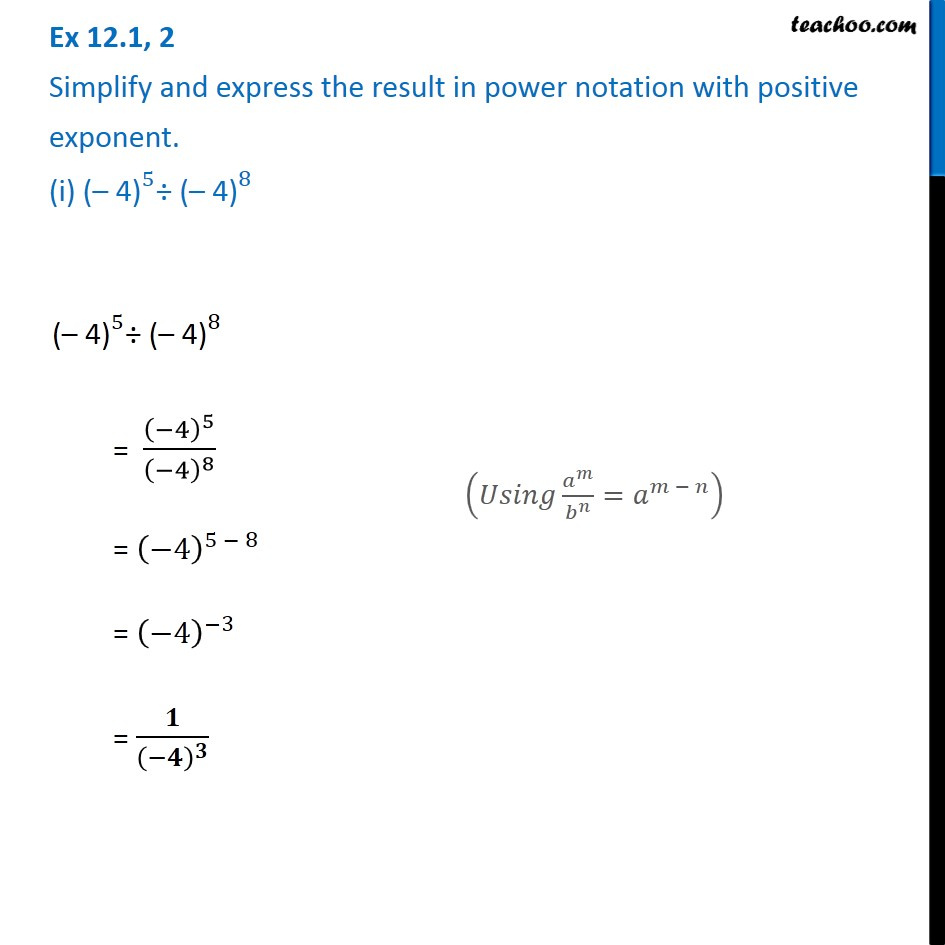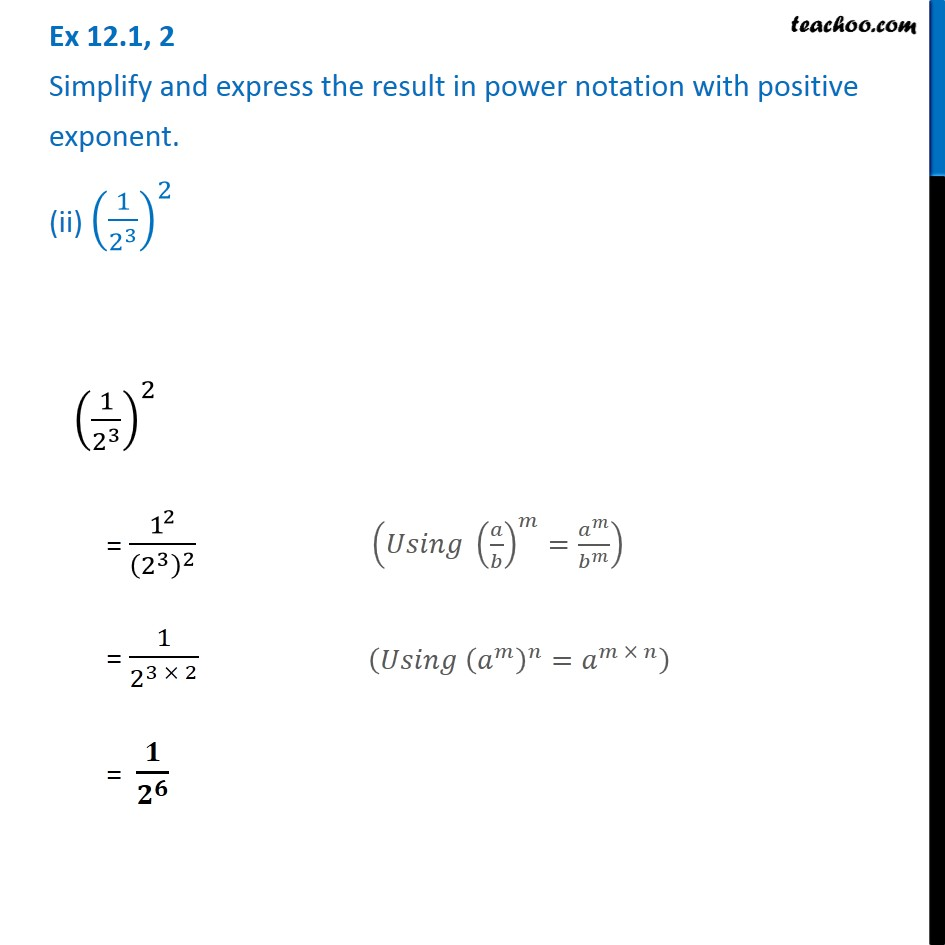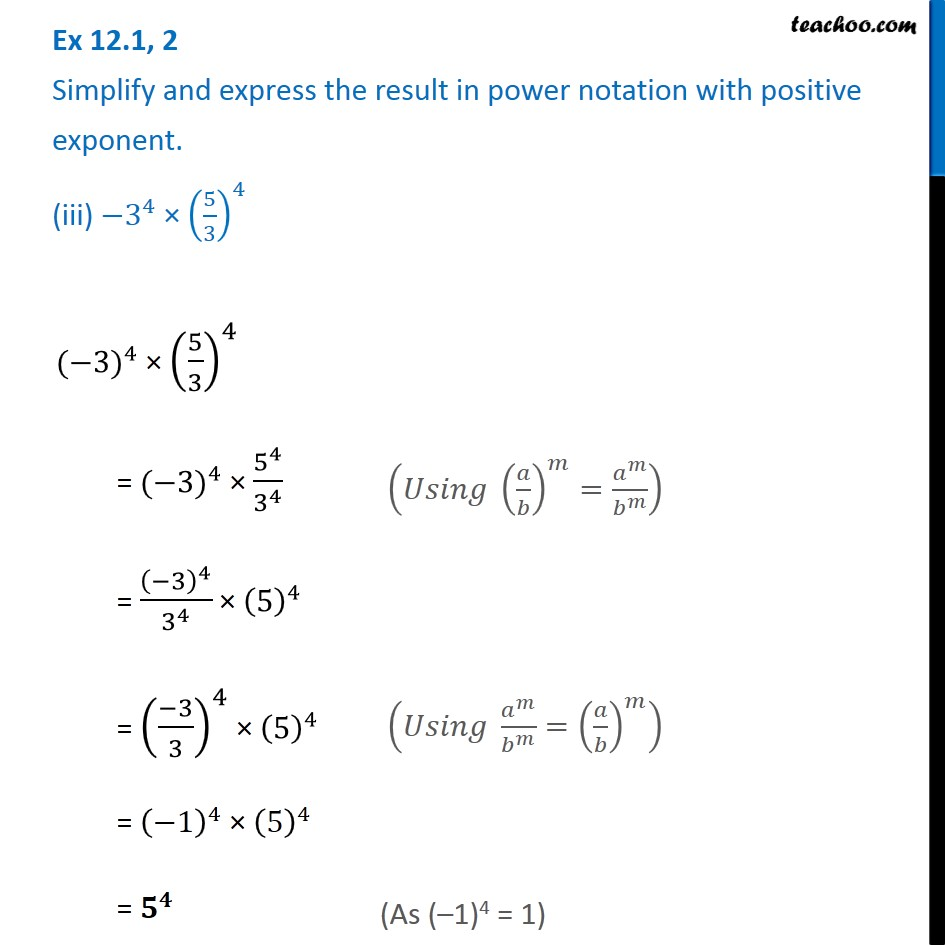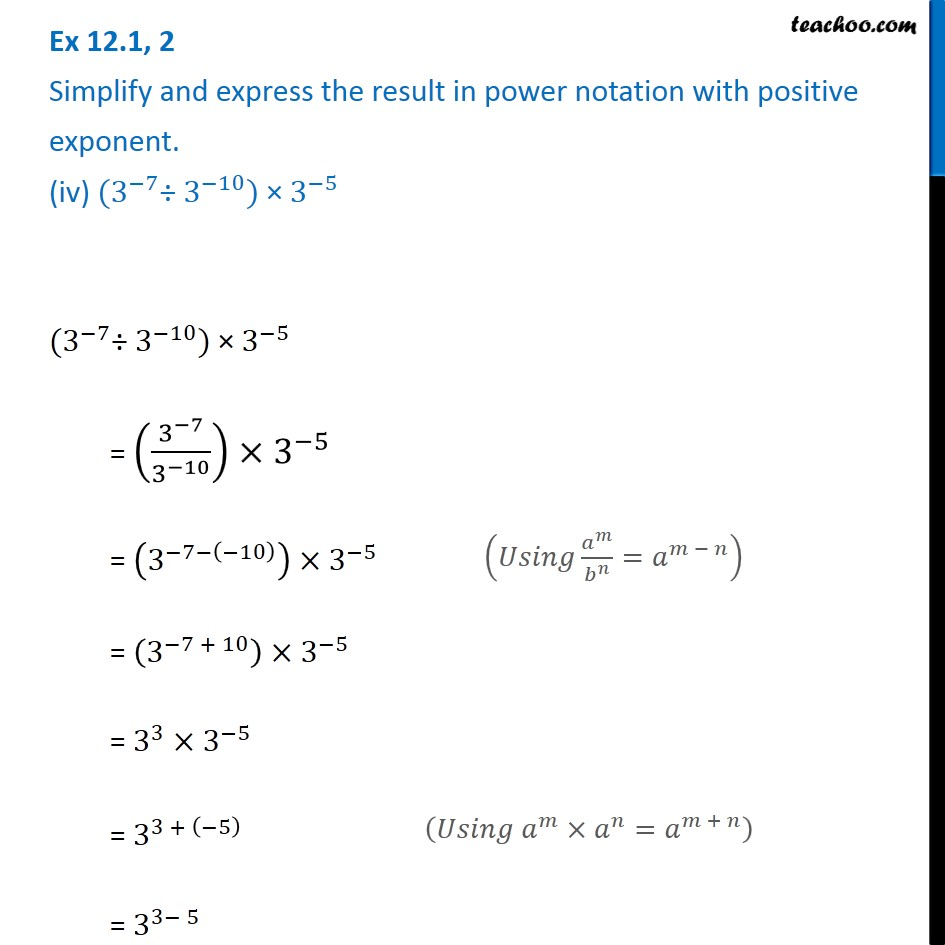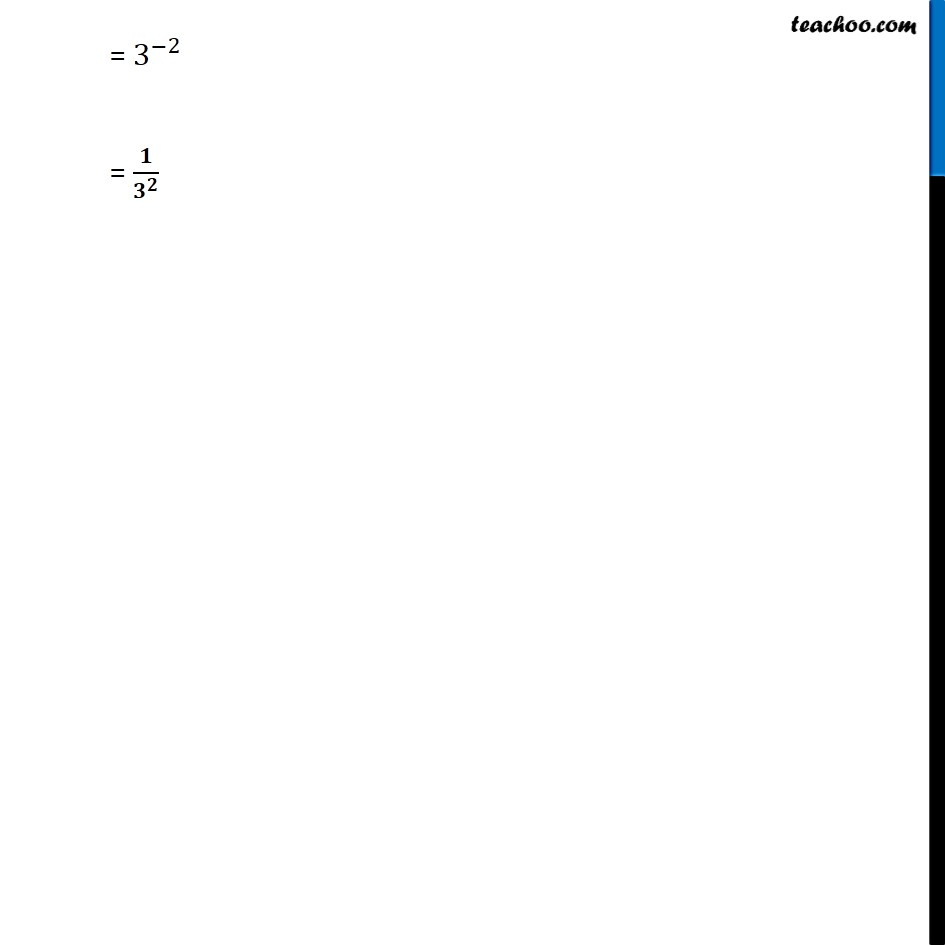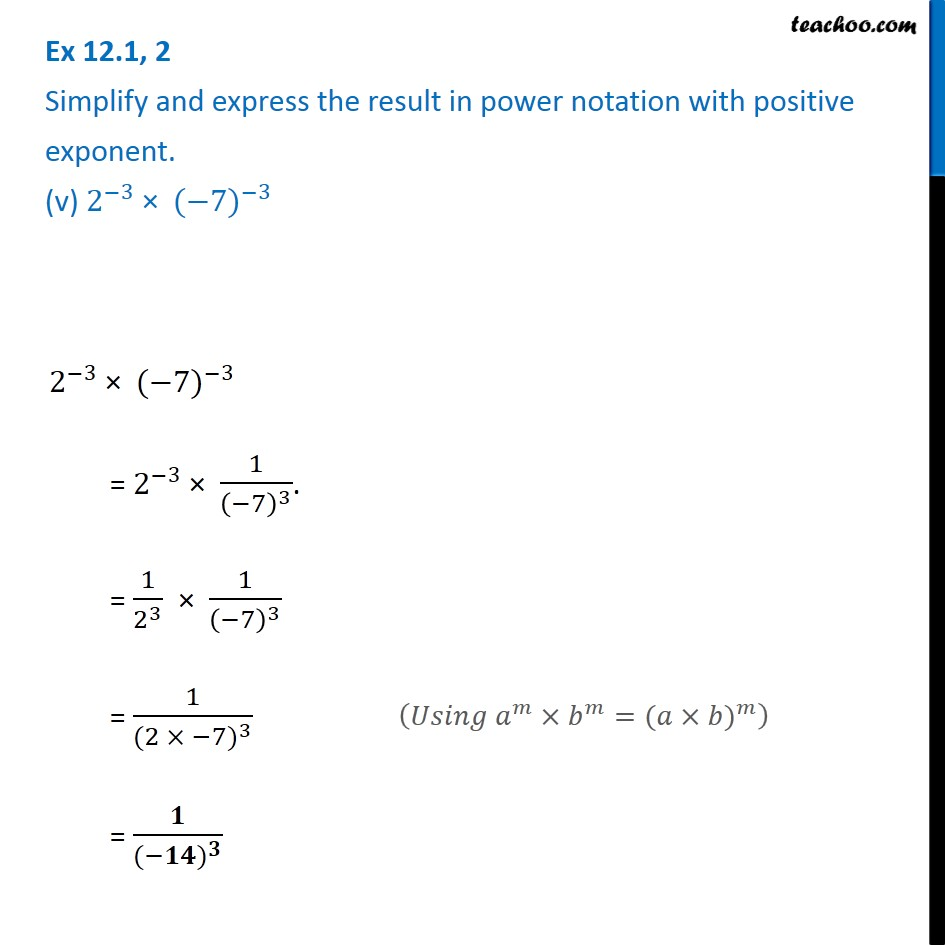Subscribe to our Youtube Channel - https://you.tube/teachoo

1. Chapter 12 Class 8 Exponents and Powers
2. Serial order wise
3. Ex 12.1

Transcript

Ex 12.1, 2 Simplify and express the result in power notation with positive exponent. (i) 〖"(– 4)" 〗^5÷ 〖"(– 4)" 〗^8 〖"(– 4)" 〗^5÷ 〖"(– 4)" 〗^8 = (−4)^5/(−4)^8 = (−4)^(5 − 8) = (−4)^(−3) = 𝟏/(−𝟒)^𝟑 (𝑈𝑠𝑖𝑛𝑔 𝑎^𝑚/𝑏^𝑛 =𝑎^(𝑚 − 𝑛) ) Ex 12.1, 2 Simplify and express the result in power notation with positive exponent. (ii) (1/2^3 )^2 (1/2^3 )^2 = 1^2/(2^3 )^2 = 1/2^(3 × 2) = 𝟏/𝟐^𝟔 (𝑈𝑠𝑖𝑛𝑔 (𝑎/𝑏)^𝑚=𝑎^𝑚/𝑏^𝑚 ) (𝑈𝑠𝑖𝑛𝑔 (𝑎^𝑚 )^𝑛=𝑎^(𝑚 × 𝑛) ) Ex 12.1, 2 Simplify and express the result in power notation with positive exponent. (iii) 〖−3〗^4 × (5/3)^4 〖(−3)〗^4 × (5/3)^4 = 〖(−3)〗^4 × 5^4/3^4 = (−3)^4/3^4 × (5)^4 = ((−3)/3)^4 × (5)^4 = (−1)^4 × (5)^4 = 𝟓^𝟒 (𝑈𝑠𝑖𝑛𝑔 (𝑎/𝑏)^𝑚=𝑎^𝑚/𝑏^𝑚 ) (𝑈𝑠𝑖𝑛𝑔 𝑎^𝑚/𝑏^𝑚 =(𝑎/𝑏)^𝑚 ) (As (–1)4 = 1) Ex 12.1, 2 Simplify and express the result in power notation with positive exponent. (iv) 〖(3〗^(−7)÷ 3^(−10)) × 3^(−5) 〖(3〗^(−7)÷ 3^(−10)) × 3^(−5) = (3^(−7)/3^(−10) )×3^(−5) = (3^(−7−(−10) ) )×3^(−5) = (3^(−7 + 10) )×3^(−5) = 3^3×3^(−5) = 3^(3 + (−5) ) = 3^(3− 5 ) (𝑈𝑠𝑖𝑛𝑔 𝑎^𝑚/𝑏^𝑛 =𝑎^(𝑚 − 𝑛) ) (𝑈𝑠𝑖𝑛𝑔 𝑎^𝑚×𝑎^𝑛=𝑎^(𝑚 + 𝑛) ) = 3^(−2) = 𝟏/𝟑^𝟐 Ex 12.1, 2 Simplify and express the result in power notation with positive exponent. (v) 2^(−3) × 〖(−7)〗^(−3) 2^(−3) × 〖(−7)〗^(−3) = 2^(−3) × 1/(−7)^3 . = 1/2^3 × 1/(−7)^3 = 1/((〖2 × −7)〗^3 ) = 𝟏/((〖−𝟏𝟒)〗^𝟑 ) (𝑈𝑠𝑖𝑛𝑔 𝑎^𝑚×𝑏^𝑚=〖(𝑎×𝑏)〗^𝑚 )

Ex 12.1